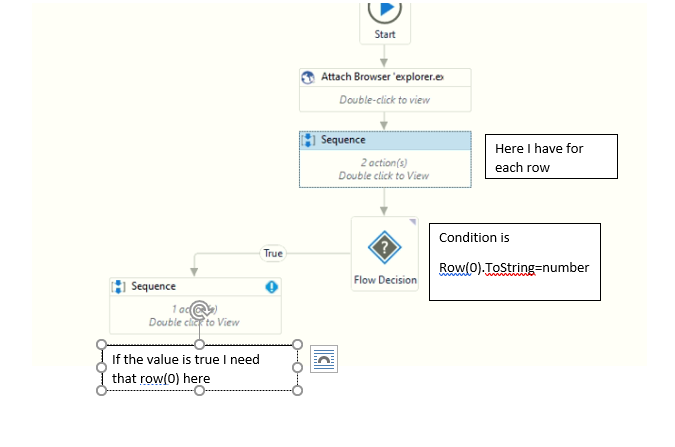# For each row to flow switch

greetings of the day…
I will get some value from the website and store it in the variable. now need to check this variable in excel for each loop the condition row(0).tostring=variable will start the next sequence. The condition is passed in the decision flow when it is true it goes fo the next sequence. Now I need to get the row values of that matched loop in this sequence is that possible.

For Eg :-As per the above img if the value that you get from web is 6 just for assumtion
So you have to check that value 6 in column Value1
If it is present der means the value from web = row(0).tostring is True
Then you should get the output for all those columns values of that matched row
As per the img you should get {6,7,8,9,10}

Right ??
This is what you are trying to do ??

Happy AutomationBest Regards
Er Pratik WavhalSomething close, Let me explain in brief. I am working with whatsapp automation at front-end. so now at first the bot will fetch the number of the new message received and will go through the excel if the number matches with excel then open that chat and reply back.
Here is the flow@soumi_soumiya

Try below in IF condition

`YourDataTable.AsEnumerable().Any(Function(x) x("columnName").ToString.ToUpper = WebVariable.ToUpper.Trim)`

Above solution will compare the variable with Webvariable and returns true or False without using For each row

Mark as solution if this helps

Thanks

3 Likes

is for each row is not necessary for this could explain in brief not able to get your point of where to implement this condition

@soumi_soumiya

You have to do for each row to compare the value one by one, but this is a LinQ Function where AsEnumerable() will work as for each row to get the values row by row in a column and it will compare with WebVariable

This is the shortest and quickest form

Mark as solution if this helps

Thanks

where to pass this LinQ function

This function you have to pass in if condition because it is comparing the Excel column value and Your Website variable value, so it will returns Boolean Value(True or False)

Mark as solution if this helps

Thanks

Yourdatatable name is the read range out right. if so then it throws an error AsEnumeranle() is not member of sstem.data.datatable

Yes, you need to put datatable, Read Range activity will output the Datatable variable you need to use that variable

Thanks

@soumi_soumiya

The error is because of missing assembly inside the code, Open Xaml file in notepad and add the string
System.Data.DataSetExtensions

as per image below

Hope this helps or you can share your xaml file, i will do that for you

Thanks

1 Like

yes used the same, read range output is customer_name so i have replaced yourdatatable with customer_name, columnname is number and webvaiable is also number
But it throws the same error

I am not sure about how to do this will attach my xml

Main.xaml (106.5 KB)

I also need to row values that matched in flow switch in my true status. Can u also help me with that

You can check attached

Main (2).xaml (106.6 KB)

Thanks

Thank you it works fine now i need the matched data from excel when condition is true how could i get it

@soumi_soumiya

Nice, Mark as solution if this helps

Thanks

@soumi_soumiya

It is already stored in your web variable, so you can use that

Thanks

sure but i need the data how could i take it out is there any possible

i need to other column value of that excel

@soumi_soumiya

Then you have try as below

Variable = Dt.Select(“[Column1]=‘text’”).ElementAt(0)(“Column2”).ToString

Hope this helps

Thanks